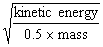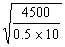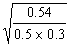QUESTIONSHEET 1

(a) work done = force ´ distance moved                                                                                                         1                         1

50  ´  10  ´  2.5                                                                                                                                        1                             1

=  1250 J/Joules                                                                                                                                       1                             1

(b) (i)      Mike    1750                                                                                                                                   1                                  1

Jeff       1500                                                                                                                             1                                  1

(ii)    Jeff                                                                                                                                                1                                       1

(iii)    Mike                                                                                                                                              1                                       1

(c) force =    work done

distance moved                                                                                                                           1                           1

1750 / 2  =  875N                                                                                                                       1                             1

875 / 10                                                                                                                                     1                                  1

87.5 kg                                                                                                                                      1                                  1

(Marks will be awarded for the calculation even if the work done by Mike was wrongly calculated in b(i))

TOTAL  / 11

QUESTIONSHEET 2

(a) work done = force ´  distance moved                                                                                                        1                         1

650  ´  500                                                                                                                                               1                                  1                                                                                                                                                   =  325 kJ/325,000 J                                                                                                                                                                       1

= 325 KJ/ 325,000 J                                                                                                                                  1

(b) 500  ´  500  =  25 000 J                                                                                                                            1                           1

250 000 / 2000                                                                                                                                          1                             1

=  125 W/watts                                                                                                                                        1                             1

(c) 650  ´  500  =  325 000 J                                                                                                                          1                           1

325 000 / 5000                                                                                                                                          1                                  1

=  65 W/watts                                                                                                                                          1                                  1

125 – 65  =  60 W/watts                                                                                                                           1                           1

Note: Do not deduct further marks if incorrect answer from b) is used correctly in subsequent calculations.

TOTAL  / 10

QUESTIONSHEET 3

(a) (i)      converted to/becomes/changes to kinetic energy.                                                                              1

(ii)   1  x  45                                                                                                                                             1                                  1

=  45 kJ/45,000 J                                                                                                                               1                             1

(b) velocity  =1

1000 / 10  =  100 kg                                                                                                                                  1

v =1

=  30 ms-1                                                                                                                                                                                                1                                            1

(c) work done = gain in potential energy                                                                                                         1                         1

=  45 kJ/45,000 J                                                                                                                                      1                             1

TOTAL  / 9

QUESTIONSHEET 4

(a) 120 ´ 10                                                                                                                                                  1                             1

=  1200 J/1.2 kJ                                                                                                                                        1                             1

(b) 120 ´  5                                                                                                                                                   1                             1

=  600 J                                                                                                                                                   1                                  1

(c) (i)      600      ´  100                                                                                                                                 1

1200

=  50%                                                                                                                                               1                                                                                                                                                                              1

(ii) friction in wheels                                                                                                                               1                             1

(iii) oil wheels                                                                                                                                         1                                  1

TOTAL  / 8

QUESTIONSHEET 5

(a) energy efficient bulb: 20 / 21  ´  100                                                                                                          1                         1

=  95%                                                                                                                                                    1                                       1

standard bulb: 100 / 180  ´  100                                                                                                                  1                         1

=  20%                                                                                                                                                    1                                       1

fluorescent strip: 20 / 28  ´  100                                                                                                                 1                         1

=  60%                                                                                                                                                    1                                       1

(b) (i)    standard bulb                                                                                                                                    1                                  1

(ii)   lost as heat                                                                                                                                       1                                  1

(c) use less electricity for the same light output                                                                                               1                    1

last longer so less resources used in a given time                                                                                        1

TOTAL  / 10

QUESTIONSHEET 6

(a) KE  =  ½  m v2                                                                                                                                                                                      1                             1

=  ½  ´  5000  ´  32                                                                                                                                                                              1                             1

=  22500 J                                                                                                                                                1                                  1

(b) 3 / 5                                                                                                                                                         1                                       1

=  0.6 m/s                                                                                                                                                1                                  1

(c) ½  ´  25 000  ´  0.62                                                                                                                                                                            1                             1

=  4500 J                                                                                                                                                  1                                  1

(d) (i)    22 500  -  4 500  =  18 000 J                                                                                                              1                           1

(ii)    converted to heat                                                                                                                              1                             1

and sound                                                                                                                                         1                                  1

TOTAL  / 10

QUESTIONSHEET 7

(a) Watts                                                                                                                                                      1                                  1

(b) one Watt  =  one Joule per second                                                                                                             1                         1

(c) energy  =  force  ´ distance                                                                                                                      1                           1

=  80  ´  10  ´  30                                                                                                                                     1                             1

=  24 000 J                                                                                                                                               1                                  1

(d) Power  =  energy / time                                                                                                                            1                           1

=  24 000 / 15                                                                                                                                           1                                  1

=  1600 W or 1.6 kW                                                                                                                                1                             1

TOTAL  / 8

QUESTIONSHEET 8

(a) 55  ´  10  =  550 N                                                                                                                                   1                             1

(b) (i)    energy  =  force  ´  distance                                                                                                              1                           1

=  3.5  ´  550                                                                                                                                    1                                  1

=  1925 J                                                                                                                                          1                                  1

(ii)    1925 J                                                                                                                                              1                                       1

(c) v2  =  u2   +  2as                                                                                                                                       1                             1

0  =  122   +  2  ´  -10  x  s                                                                                                                        1                           1

s  =  144 / 20                                                                                                                                            1                                  1

=  7.2 m                                                                                                                                                   1                                  1

(d) 900 / 3                                                                                                                                                     1                                       1

=  300 W                                                                                                                                                 1                                  1

TOTAL  / 11

QUESTIONSHEET 9

(a) (i)   30 ´ 10 ´ 3                                                                                                                                       1                                  1

=  900 J/joules                                                                                                                                 1                                  1

(b) 900 J/joules                                                                                                                                              1                                  1

(c) (1)   muscles of the man/food man has eaten/

chemical energy in food                                                                                                                       1                           1

(2) lift motor                                                                                                                                            1                                  1

TOTAL  / 5

QUESTIONSHEET 10

(a) (i)      600 kJ/600,000 J                                                                                                                             1                             1

(ii)   3.6 MJ/3,600 kJ                                                                                                                                1                             1

(b) energy of light bulb  =  1 500 000 W                                                                                                          1                         1

time  =  energy transferred / power                                                                                                           1                         1

4 h 10 min/250 min                                                                                                                                   1                             1

(c) (i)      electrical to heat energy                                                                                                                  1                           1

(ii)      electrical to heat and light energy                                                                                                1                         1

(iii)   electrical to heat and movement/kinetic energy                                                                                   1

TOTAL  / 8

QUESTIONSHEET 11

(a) (i)      potential energy to kinetic energy                                                                                                     1                         1

(ii)   kinetic energy to sound and kinetic energy                                                                                          1                    1

(iii)    kinetic energy to potential energy                                                                                                       1                         1

(b) (i)    300 / 1000  ´  10                                                                                                                               1                             1

=  0.3  ´  1.8                                                                                                                                     1                                  1

=  0.54 J                                                                                                                                         1                                  1

(ii)   potential energy has become kinetic energy at the instant

it hits the ground                                                                                                                               1                             1   =  SQRT (o.54  /  0.5  x  0.3)                                                                                                                                1

=1

=  6 ms-1                                                                                                                                                                                         1                                            1

TOTAL  / 9

QUESTIONSHEET 12

(a) using work done = force ´ distance moved                                                                                                1                                  1

430 ´ 4/4 = 430 W  (Lucy)                                                                                                                       1                                  1

450 ´ 4/6 = 300 W (Carol)                                                                                                                         1                                  1

(b) Lucy                                                                                                                                                       1                                  1

(c) her muscles                                                                                                                                             1                                  1

(d) Carol                                                                                                                                                       1                                  1

She would do the most work as she was the heaviest/ had greatest weight                                                   1                             1

TOTAL  / 7

QUESTIONSHEET 13

(a) supplied as part of nature/generated in a way that the Earth’s resources not used up.                                   1                           1

(b) (i)      200                                                                                                                                                1                                       1

(ii)    Wind does not blow all the time.                                                                                                         1                         1

(c) (i)      rearranging equation                                                                                                                        1                             1

5  ´  3300  ´  60                                                                                                                               1                             1

=  990,000 M J                                                                                                                                  1                             1

(ii)    495,000 MJ                                                                                                                                       1                                  1

TOTAL  / 7

QUESTIONSHEET 14

(a) Joule                                                                                                                                                        1                                       1

(b) (i)    energy  =  force  ´  distance                                                                                                              1                           1

0.3  ´  10  ´  0.5                                                                                                                               1                             1

1.5 J                                                                                                                                                 1                                       1

(ii)    1.5 J                                                                                                                                                 1                                       1

(iii)   0.3  ´  10                                                                                                                                          1                                  1

3 N                                                                                                                                                  1                                       1

TOTAL  / 7

QUESTIONSHEET 15

(a) (i)    No loss of kinetic energy                                                                                                                   1                           1

(ii)    lose energy as heat                                                                                                                           1                             1

and sound                                                                                                                                         1                                  1

(b) (Two from)

air bag - absorbs kinetic energy of driver

crumple zone – absorbs KE of car

seat belt – exerts force to stop driver                                                                                                         4                         4

(c) (i)    KE =  ½ m v2                                                                                                                                                                              1                                  1

=  ½  ´  2000  ´  152                                                                                                                                                        1                             1

=  225 000 J  or  225 kJ                                                                                                              1                           1

(ii)    Force   =  energy / distance                                                                                                               1                           1

=  225 000 / 30                                                                                                                      1                             1

=  7500 N                                                                                                                             1                                  1

TOTAL  / 13

QUESTIONSHEET 16

(a) (i)    chemical                                                                                                                                           1                                  1

à  electrical                                                                                                                                     1                                   1

à  kinetic                                                                                                                                        1                                  1

(ii)    work done  =  force  x distance                                                                                                          1                         1

=  3  ´  1  =  3 J                                                                                                                                1                             1

(iii)   Power  =  energy / time                                                                                                                     1                           1

=  3 / 10   =   0.3 W                                                                                                                          1                             1

(b) (i)    potential                                                                                                                                           1                                  1

à  kinetic                                                                                                                                        1                                  1

à  electrical                                                                                                                                     1                                  1

à  heat  &  light                                                                                                                               2                             2

(ii)    KE =  ½ m v2                                                                                                                                                                              1                                  1

=  ½  ´  1 ´  22                                                                                                                                                                   1                             1                                                                                                                                             =  2 J                                       1

(c) chemical                                                                                                                                                  1                                  1

à  heat                                                                                                                                                   1                                  1

à  kinetic                                                                                                                                                1                                  1

TOTAL  / 17

QUESTIONSHEET 17

(a) heat and light  (either order)                                                                                                                      2                           2

(b) chemical                                                                                                                                                  1                                  1

sound                                                                                                                                                       1                                  1

(c) kinetic and sound  (either order)                                                                                                                2                    2

(d) heat and light  (either order)                                                                                                                      2                         2

TOTAL  / 8

QUESTIONSHEET 18

(a) Six from

kinetic, potential, electrical, heat, light, sound,

chemical, nuclear                                                                                                                                      6                             6

(b) (i)    Newton                                                                                                                                            1                                  1

metre                                                                                                                                               1                                       1

(ii)    conservation                                                                                                                                     1                                  1

changed                                                                                                                                           1                                  1

created                                                                                                                                             1                                       1

destroyed                                                                                                                                         1                                  1

TOTAL  / 12

QUESTIONSHEET 19

(a) too much emphasis on nuclear energy                                                                                                        1                    1

not enough spent on renewable sources                                                                                                     1                    1

(b) secondary source of energy                                                                                                                      1                           1

made from the others                                                                                                                                1                             1

(c) gas                                                                                                                                                          1                                       1

oil                                                                                                                                                            1                                  1

TOTAL  / 6

QUESTIONSHEET 20

(a) (i)    the sun                                                                                                                                             1                                       1

(ii)    photosynthesis                                                                                                                                  1                                  1

produces glucose / sugar which stores energy                                                                                     1               1            converted to storable form                                                                                                                                           1

(iii)   plants die                                                                                                                                          1                                  1

subjected to high temperatures and pressures                                                                                      1                    1

over millions of years                                                                                                                        1                             1

(b) (i)    A                                                                                                                                                     1                                  1

(ii)    C                                                                                                                                                     1                                  1

TOTAL  / 8